Open In App

# Learn Data Structures with Javascript | DSA Tutorial

JavaScript (JS) is the most popular lightweight, interpreted compiled programming language, and might be your first preference for Client-side as well as Server-side developments. But have you thought about using Javascript for DSA? Learning Data Structures and Algorithms can be difficult when combined with Javascript. For this reason, we have brought to you this detailed DSA tutorial on how to get started with Data Structures with Javascript from scratch.

## What is Data Structure?

A data structure is defined as a particular way of storing and organizing data in our devices to use the data efficiently and effectively. The main idea behind using data structures is to minimize the time and space complexities. An efficient data structure takes minimum memory space and requires minimum time to execute the data.Data Structures with JavascriptData Structures with Javascript

## How to start learning Data Structures?

The first and foremost thing is dividing the total procedure into little pieces which need to be done sequentially.

The complete process to learn DS from scratch can be broken into 3 parts:

1. Learn about Time and Space complexities
2. Learn the basics of individual Data Structures
3. Practice Problems on Data Structures

Here comes one of the interesting and important topics. The primary motive to use DSA is to solve a problem effectively and efficiently. How can you decide if a program written by you is efficient or not? This is measured by complexities. Complexity is of two types:

1. Time Complexity: Time complexity is used to measure the amount of time required to execute the code.
2. Space Complexity: Space complexity means the amount of space required to execute successfully the functionalities of the code.
You will also come across the term Auxiliary Space very commonly in DSA, which refers to the extra space used in the program other than the input data structure.

Both of the above complexities are measured with respect to the input parameters. But here arises a problem. The time required for executing a code depends on several factors, such as:

• The number of operations performed in the program,
• The speed of the device, and also
• The speed of data transfer is being executed on an online platform.

## 2. Learn Data Structures

Here comes the most crucial and the most awaited stage of the roadmap for learning data structure and algorithm – the stage where you start learning about DSA. The topic of DSA consists of two parts:

• Data Structures
• Algorithms

Though they are two different things, they are highly interrelated, and it is very important to follow the right track to learn them most efficiently. If you are confused about which one to learn first, we recommend you to go through our detailed analysis on the topic: What should I learn first- Data Structures or Algorithms?

Here we have followed the flow of learning a data structure and then the most related and important algorithms used by that data structure.

### 1. Array in javascript

An array is a collection of items of the same variable type stored that are stored at contiguous memory locations. It’s one of the most popular and simple data structures and is often used to implement other data structures. Each item in an array is indexed starting with 0.

Declaration of an Array: There are basically two ways to declare an array.

Syntax:

```let arrayName = [value1, value2, ...]; // Method 1
let arrayName = new Array(); // Method 2```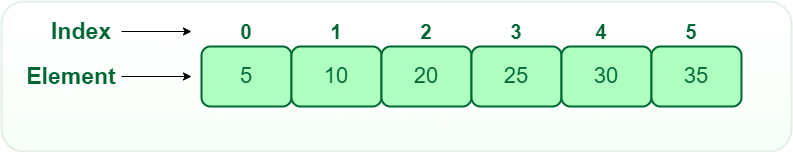Array Data Structure

Types of Array operations:

• Traversal: Traverse through the elements of an array.
• Insertion: Inserting a new element in an array.
• Deletion: Deleting element from the array.
• Searching:  Search for an element in the array.
• Sorting: Maintaining the order of elements in the array.

Below is the implementation of the array in javascript:

## Javascript

 `// Initializing while declaring``    ``// Creates an array having elements 10, 20, 30, 40, 50``    ``var` `house = ``new` `Array(10, 20, 30, 40, 50);``    ` `    ``// Creates an array of 5 undefined elements``    ``var` `house1 = ``new` `Array(5);``    ` `    ``// Creates an array with element 1BHK``    ``var` `home = ``new` `Array(``"1BHK"``);``    ``console.log(house)``    ``console.log(house1)``    ``console.log(home)`

Output

```[ 10, 20, 30, 40, 50 ]
[ <5 empty items> ]
[ '1BHK' ]```

### 2. String in javascript

JavaScript strings are used for storing and manipulating text. It can contain zero or more characters within quotes.

Creating Strings: There are two ways to create a string in Javascript:

• By string literal
• By string object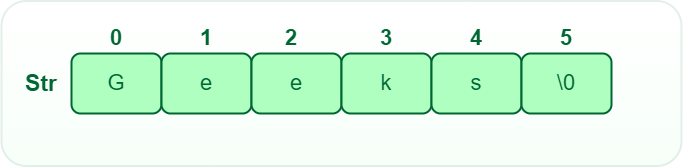String Data Structure

String operations:

• Substrings:  A substring is a contiguous sequence of characters within a string
• Concatenation: This operation is used for appending one string to the end of another string.
• Length: It defines the number of characters in the given string.
• Text Processing Operations: Text processing is the process of creating and editing strings.
• Insertion: This operation is used to insert characters in the string at the specified position.
• Deletion: This operation is used to delete characters in the string at the specified position.
• Update: This operation is used to update characters in the string at the specified position.

Below is the implementation of the String in javascript:

## Javascript

 `// String written inside quotes``    ``var` `x = ``"Welcome to GeeksforGeeks!"``;``    ``console.log(x);``    ` `      ``// Declare an object``    ``var` `y = ``new` `String(``"Great Geek"``);``   ``console.log(y);``   ` `   ` `    ``let a = ``"abcdefgh"``;` `    ``// Finding the first index of the character 'b'``    ``console.log(a.indexOf(``'b'``));`  `    ``let a2 = ``"Hello World"``;` `    ``let arrString = [``"Geeks"``, ``"for"``, ``"Geeks"``]` `    ``// Replacing the word 'World' with 'Geeks'``    ``console.log(a2.replace(``"World"``, arrString));`

Output

```Welcome to GeeksforGeeks!
[String: 'Great Geek']
1
Hello Geeks```

### 3.  Linked List in Javascript

A linked list is a linear data structure, Unlike arrays, linked list elements are not stored at a contiguous location. it is basically chains of nodes, each node contains information such as data and a pointer to the next node in the chain. In the linked list there is a head pointer, which points to the first element of the linked list, and if the list is empty then it simply points to null or nothing.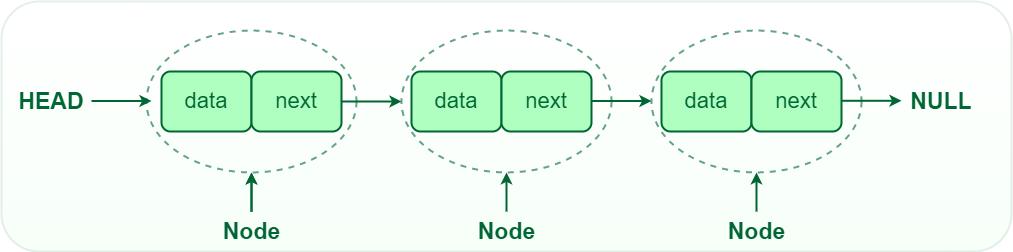• Traversal: We can traverse the entire linked list starting from the head node. If there are n nodes then the time complexity for traversal becomes O(n) as we hop through each and every node.
• Insertion: Insert a key to the linked list. An insertion can be done in 3 different ways; insert at the beginning of the list, insert at the end of the list and insert in the middle of the list.
• Deletion: Removes an element x from a given linked list. You cannot delete a node by a single step. A deletion can be done in 3 different ways; delete from the beginning of the list, delete from the end of the list and delete from the middle of the list.
• Search: Find the first element with the key k in the given linked list by a simple linear search and returns a pointer to this element

Below is the implementation of the Linked list  in javascript:

## Javascript

 `class Node {``    ``// constructor``    ``constructor(element) {``        ``this``.element = element;``        ``this``.next = ``null``    ``}``}``// linkedlist class``class LinkedList {``    ``constructor() {``        ``this``.head = ``null``;``        ``this``.size = 0;``    ``}` `    ``// adds an element at the end``    ``// of list``    ``add(element) {``        ``// creates a new node``        ``var` `node = ``new` `Node(element);` `        ``// to store current node``        ``var` `current;` `        ``// if list is Empty add the``        ``// element and make it head``        ``if` `(``this``.head == ``null``)``            ``this``.head = node;``        ``else` `{``            ``current = ``this``.head;` `            ``// iterate to the end of the``            ``// list``            ``while` `(current.next) {``                ``current = current.next;``            ``}` `            ``// add node``            ``current.next = node;``        ``}``        ``this``.size++;``    ``}` `    ``// insert element at the position index``    ``// of the list``    ``insertAt(element, index) {``        ``if` `(index < 0 || index > ``this``.size)``            ``return` `console.log(``"Please enter a valid index."``);``        ``else` `{``            ``// creates a new node``            ``var` `node = ``new` `Node(element);``            ``var` `curr, prev;` `            ``curr = ``this``.head;` `            ``// add the element to the``            ``// first index``            ``if` `(index == 0) {``                ``node.next = ``this``.head;``                ``this``.head = node;``            ``} ``else` `{``                ``curr = ``this``.head;``                ``var` `it = 0;` `                ``// iterate over the list to find``                ``// the position to insert``                ``while` `(it < index) {``                    ``it++;``                    ``prev = curr;``                    ``curr = curr.next;``                ``}` `                ``// adding an element``                ``node.next = curr;``                ``prev.next = node;``            ``}``            ``this``.size++;``        ``}``    ``}` `    ``// removes an element from the``    ``// specified location``    ``removeFrom(index) {``        ``if` `(index < 0 || index >= ``this``.size)``            ``return` `console.log(``"Please Enter a valid index"``);``        ``else` `{``            ``var` `curr, prev, it = 0;``            ``curr = ``this``.head;``            ``prev = curr;` `            ``// deleting first element``            ``if` `(index === 0) {``                ``this``.head = curr.next;``            ``} ``else` `{``                ``// iterate over the list to the``                ``// position to removce an element``                ``while` `(it < index) {``                    ``it++;``                    ``prev = curr;``                    ``curr = curr.next;``                ``}` `                ``// remove the element``                ``prev.next = curr.next;``            ``}``            ``this``.size--;` `            ``// return the remove element``            ``return` `curr.element;``        ``}``    ``}` `    ``// removes a given element from the``    ``// list``    ``removeElement(element) {``        ``var` `current = ``this``.head;``        ``var` `prev = ``null``;` `        ``// iterate over the list``        ``while` `(current != ``null``) {``            ``// comparing element with current``            ``// element if found then remove the``            ``// and return true``            ``if` `(current.element === element) {``                ``if` `(prev == ``null``) {``                    ``this``.head = current.next;``                ``} ``else` `{``                    ``prev.next = current.next;``                ``}``                ``this``.size--;``                ``return` `current.element;``            ``}``            ``prev = current;``            ``current = current.next;``        ``}``        ``return` `-1;``    ``}`  `    ``// finds the index of element``    ``indexOf(element) {``        ``var` `count = 0;``        ``var` `current = ``this``.head;` `        ``// iterate over the list``        ``while` `(current != ``null``) {``            ``// compare each element of the list``            ``// with given element``            ``if` `(current.element === element)``                ``return` `count;``            ``count++;``            ``current = current.next;``        ``}` `        ``// not found``        ``return` `-1;``    ``}` `    ``// checks the list for empty``    ``isEmpty() {``        ``return` `this``.size == 0;``    ``}` `    ``// gives the size of the list``    ``size_of_list() {``        ``console.log(``this``.size);``    ``}`  `    ``// prints the list items``    ``printList() {``        ``var` `curr = ``this``.head;``        ``var` `str = ``""``;``        ``while` `(curr) {``            ``str += curr.element + ``" "``;``            ``curr = curr.next;``        ``}``        ``console.log(str);``    ``}` `}` `// creating an object for the``// Linkedlist class``var` `ll = ``new` `LinkedList();` `// testing isEmpty on an empty list``// returns true``console.log(ll.isEmpty());` `// adding element to the list``ll.add(10);` `// prints 10``ll.printList();` `// returns 1``console.log(ll.size_of_list());` `// adding more elements to the list``ll.add(20);``ll.add(30);``ll.add(40);``ll.add(50);` `// returns 10 20 30 40 50``ll.printList();` `// prints 50 from the list``console.log(``"is element removed ?"` `+ ll.removeElement(50));` `// prints 10 20 30 40``ll.printList();` `// returns 3``console.log(``"Index of 40 "` `+ ll.indexOf(40));` `// insert 60 at second position``// ll contains 10 20 60 30 40``ll.insertAt(60, 2);` `ll.printList();` `// returns false``console.log(``"is List Empty ? "` `+ ll.isEmpty());` `// remove 3rd element from the list``console.log(ll.removeFrom(3));` `// prints 10 20 60 40``ll.printList();`

Output

```true
10
1
undefined
10 20 30 40 50
is element removed ?50
10 20 30 40
Index of 40 3
10 20 60 30 40
is List Empty ? false
30
10 20 60 40 ```

### 4. Stack in Javascript

Stack is a linear data structure in which insertion and deletion are done at one end this end is generally called the top. It works on the principle of Last In First Out (LIFO) or First in Last out (FILO). LIFO means the last element inserted inside the stack is removed first. FILO means, the last inserted element is available first and is the first one to be deleted.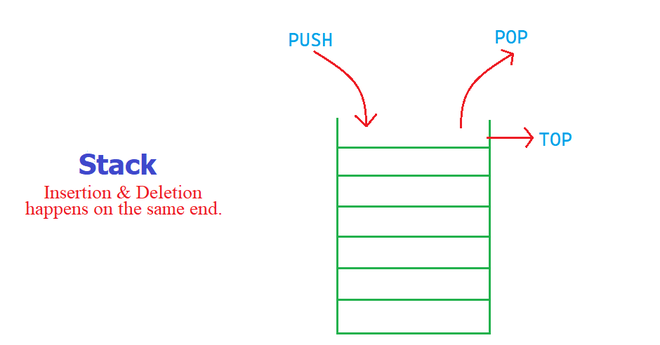Stack Data structure

Operations in a Stack:

1. Push: Add an element to the top of a stack
2. Pop: Remove an element from the top of a stack
3. IsEmpty: Check if the stack is empty
4. IsFull: Check if the stack is full
5. top/Peek: Get the value of the top element without removing it

Below is the implementation of the Stack  in javascript:

## Javascript

 `// Stack class``class Stack {` `    ``// Array is used to implement stack``    ``constructor()``    ``{``        ``this``.items = [];``    ``}` `    ``// Functions to be implemented``    ``// push(item)``    ``// push function``  ``push(element)``{``    ``// push element into the items``    ``this``.items.push(element);``}` `    ` `    ``// pop function``pop()``{``    ``// return top most element in the stack``    ``// and removes it from the stack``    ``// Underflow if stack is empty``    ``if` `(``this``.items.length == 0)``        ``return` `"Underflow"``;``    ``return` `this``.items.pop();``}` `// peek function`` ``peek()``{``    ``// return the top most element from the stack``    ``// but does'nt delete it.``    ``return` `this``.items[``this``.items.length - 1];``}`  ` ``// isEmpty function``  ``isEmpty()``{``    ``// return true if stack is empty``    ``return` `this``.items.length == 0;``}` `    ` `    ``// printStack function`` ``printStack()``{``    ``var` `str = ``""``;``    ``for` `(``var` `i = 0; i < ``this``.items.length; i++)``        ``str += ``this``.items[i] + ``" "``;``    ``return` `str;``}` `}``// creating object for stack class``var` `stack = ``new` `Stack();` `// testing isEmpty and pop on an empty stack` `// returns false``console.log(stack.isEmpty());` `// returns Underflow``console.log(stack.pop());``// Adding element to the stack``stack.push(10);``stack.push(20);``stack.push(30);` `// Printing the stack element``// prints [10, 20, 30]``console.log(stack.printStack());` `// returns 30``console.log(stack.peek());` `// returns 30 and remove it from stack``console.log(stack.pop());` `// returns [10, 20]``console.log(stack.printStack());`

Output

```true
Underflow
10 20 30
30
30
10 20 ```

### 5. Queue in Javascript

A Queue is a linear structure that follows a particular order in which the operations are performed. The order is First In First Out (FIFO). It is similar to the ticket queue outside a cinema hall, where the first person entering the queue is the first person who gets the ticket.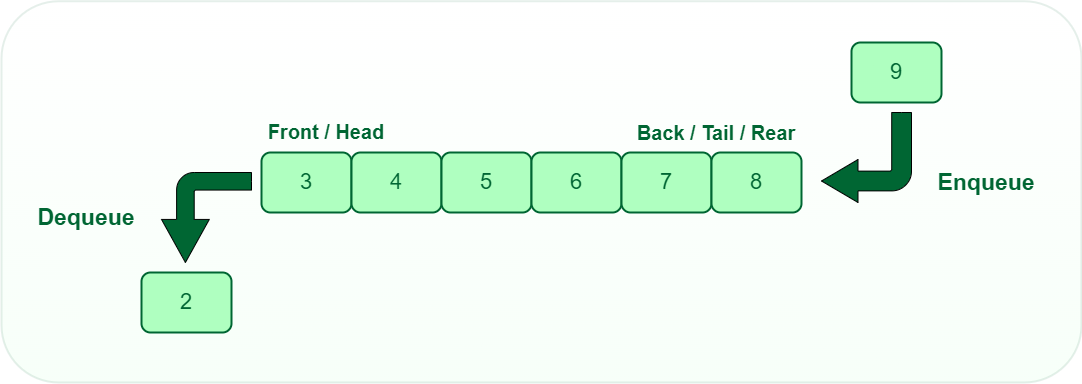Queue Data Structure

Operations of Queue:

A queue is an object (an abstract data structure – ADT) that allows the following operations:

1. Enqueue: Add an element to the end of the queue
2. Dequeue: Remove an element from the front of the queue
3. IsEmpty: Check if the queue is empty
4. IsFull: Check if the queue is full
5. top/Peek: Get the value of the front of the queue without removing it

Below is the implementation of Queue in javascript:

## Javascript

 `class Queue {``        ``constructor() {``            ``this``.items = {}``            ``this``.frontIndex = 0``            ``this``.backIndex = 0``        ``}``        ``enqueue(item) {``            ``this``.items[``this``.backIndex] = item``            ``this``.backIndex++``            ``return` `item + ``' inserted'``        ``}``        ``dequeue() {``            ``const item = ``this``.items[``this``.frontIndex]``            ``delete` `this``.items[``this``.frontIndex]``            ``this``.frontIndex++``            ``return` `item``        ``}``        ``peek() {``            ``return` `this``.items[``this``.frontIndex]``        ``}``        ``get printQueue() {``            ``return` `this``.items;``        ``}``        ``// isEmpty function``isEmpty() {``    ``// return true if the queue is empty.``    ``return` `this``.items.length == 0;``}` `    ``}``    ``const queue = ``new` `Queue()``    ``console.log(queue.enqueue(7))``    ``console.log(queue.enqueue(2))``    ``console.log(queue.enqueue(6))``    ``console.log(queue.enqueue(4))``    ``console.log(queue.dequeue())``    ``console.log(queue.peek())``    ``var` `str = queue.printQueue;``    ``console.log(str)`

Output

```7 inserted
2 inserted
6 inserted
4 inserted
7
2
{ '1': 2, '2': 6, '3': 4 }```

### 6. Tree in Javascript

A tree is non-linear and has a hierarchical data structure consisting of a collection of nodes such that each node of the tree stores a value and a list of references to other nodes (the “children”).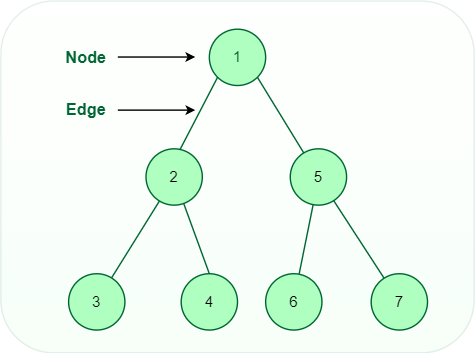Tree Data Structure

Types of Trees:

Operations on tree data structure:

• Insert: Insert an element in a tree/create a tree.
• Search: Searches an element in a tree.
• Tree Traversal: The tree traversal algorithm is used in order to visit a specific node in the tree to perform a specific operation on it.

Below is the implementation of Binary Search Tree in javascript:

## Javascript

 `// Node class``class Node``{``    ``constructor(data)``    ``{``        ``this``.data = data;``        ``this``.left = ``null``;``        ``this``.right = ``null``;``    ``}``}``// Binary Search tree class``class BinarySearchTree``{``    ``constructor()``    ``{``        ``// root of a binary search tree``        ``this``.root = ``null``;``    ``}` `    ``// function to be implemented``    ``// helper method which creates a new node to``// be inserted and calls insertNode``insert(data)``{``    ``// Creating a node and initialising``    ``// with data``    ``var` `newNode = ``new` `Node(data);``                    ` `    ``// root is null then node will``    ``// be added to the tree and made root.``    ``if``(``this``.root === ``null``)``        ``this``.root = newNode;``    ``else` `        ``// find the correct position in the``        ``// tree and add the node``        ``this``.insertNode(``this``.root, newNode);``}` `// Method to insert a node in a tree``// it moves over the tree to find the location``// to insert a node with a given data``insertNode(node, newNode)``{``    ``// if the data is less than the node``    ``// data move left of the tree``    ``if``(newNode.data < node.data)``    ``{``        ``// if left is null insert node here``        ``if``(node.left === ``null``)``            ``node.left = newNode;``        ``else` `            ``// if left is not null recur until``            ``// null is found``            ``this``.insertNode(node.left, newNode);``    ``}` `    ``// if the data is more than the node``    ``// data move right of the tree``    ``else``    ``{``        ``// if right is null insert node here``        ``if``(node.right === ``null``)``            ``node.right = newNode;``        ``else` `            ``// if right is not null recur until``            ``// null is found``            ``this``.insertNode(node.right, newNode);``    ``}``}` `    ``// helper method that calls the``// removeNode with a given data``remove(data)``{``    ``// root is re-initialized with``    ``// root of a modified tree.``    ``this``.root = ``this``.removeNode(``this``.root, data);``}` `// Method to remove node with a``// given data``// it recur over the tree to find the``// data and removes it``removeNode(node, key)``{``        ` `    ``// if the root is null then tree is``    ``// empty``    ``if``(node === ``null``)``        ``return` `null``;` `    ``// if data to be delete is less than``    ``// roots data then move to left subtree``    ``else` `if``(key < node.data)``    ``{``        ``node.left = ``this``.removeNode(node.left, key);``        ``return` `node;``    ``}` `    ``// if data to be delete is greater than``    ``// roots data then move to right subtree``    ``else` `if``(key > node.data)``    ``{``        ``node.right = ``this``.removeNode(node.right, key);``        ``return` `node;``    ``}` `    ``// if data is similar to the root's data``    ``// then delete this node``    ``else``    ``{``        ``// deleting node with no children``        ``if``(node.left === ``null` `&& node.right === ``null``)``        ``{``            ``node = ``null``;``            ``return` `node;``        ``}` `        ``// deleting node with one children``        ``if``(node.left === ``null``)``        ``{``            ``node = node.right;``            ``return` `node;``        ``}``        ` `        ``else` `if``(node.right === ``null``)``        ``{``            ``node = node.left;``            ``return` `node;``        ``}` `        ``// Deleting node with two children``        ``// minimum node of the right subtree``        ``// is stored in aux``        ``var` `aux = ``this``.findMinNode(node.right);``        ``node.data = aux.data;` `        ``node.right = ``this``.removeNode(node.right, aux.data);``        ``return` `node;``    ``}` `}` `                `  `    ` `    ``// finds the minimum node in tree``// searching starts from given node``findMinNode(node)``{``    ``// if left of a node is null``    ``// then it must be minimum node``    ``if``(node.left === ``null``)``        ``return` `node;``    ``else``        ``return` `this``.findMinNode(node.left);``}` `    ` `    ``// returns root of the tree``getRootNode()``{``    ``return` `this``.root;``}`  `    ``// Performs inorder traversal of a tree``inorder(node)``{``    ``if``(node !== ``null``)``    ``{``        ``this``.inorder(node.left);``        ``console.log(node.data);``        ``this``.inorder(node.right);``    ``}``}` `    ``// Performs preorder traversal of a tree``preorder(node)``{``    ``if``(node !== ``null``)``    ``{``        ``console.log(node.data);``        ``this``.preorder(node.left);``        ``this``.preorder(node.right);``    ``}``}` `    ` `// Performs postorder traversal of a tree``postorder(node)``{``    ``if``(node !== ``null``)``    ``{``        ``this``.postorder(node.left);``        ``this``.postorder(node.right);``        ``console.log(node.data);``    ``}``}` `    ` `    ``// search for a node with given data``search(node, data)``{``// if trees is empty return null``    ``if``(node === ``null``)``        ``return` `null``;` `    ``// if data is less than node's data``    ``// move left``    ``else` `if``(data < node.data)``        ``return` `this``.search(node.left, data);` `    ``// if data is more than node's data``    ``// move right``    ``else` `if``(data > node.data)``        ``return` `this``.search(node.right, data);` `    ``// if data is equal to the node data``    ``// return node``    ``else``        ``return` `node;``}` `    ` `}``// create an object for the BinarySearchTree``var` `BST = ``new` `BinarySearchTree();` `// Inserting nodes to the BinarySearchTree``BST.insert(15);``BST.insert(25);``BST.insert(10);``BST.insert(7);``BST.insert(22);``BST.insert(17);``BST.insert(13);``BST.insert(5);``BST.insert(9);``BST.insert(27);``                        ` `//        15``//      /   \``//     10    25``//     / \   / \``//    7   13 22 27``// / \  /``// 5 9 17` `var` `root = BST.getRootNode();``            ` `// prints 5 7 9 10 13 15 17 22 25 27``console.log(``"Initial tree: "``);``BST.inorder(root);``            ` `// Removing node with no children``BST.remove(5);` `//        15``//      /   \``//     10    25``//     / \   / \``//    7   13 22 27``//   \  /``//   9 17``                        ` `var` `root = BST.getRootNode();``            ` `console.log(``"Tree after removing 5: "``);``// prints 7 9 10 13 15 17 22 25 27``BST.inorder(root);``            ` `// Removing node with one child``BST.remove(7);``            ` `//         15``//         / \``//     10 25``//     / \ / \``//     9 13 22 27``//         /``//         17``            ` `            ` `var` `root = BST.getRootNode();` `console.log(``"Tree after removing 7: "``);``// prints 9 10 13 15 17 22 25 27``BST.inorder(root);``            ` `// Removing node with two children``BST.remove(15);``    ` `//         17``//         / \``//     10 25``//     / \ / \``//     9 13 22 27` `var` `root = BST.getRootNode();``console.log(``"Inorder traversal: "``);``// prints 9 10 13 17 22 25 27``BST.inorder(root);``            ` `console.log(``"Postorder traversal: "``);``BST.postorder(root);` `console.log(``"Preorder traversal: "``);``BST.preorder(root);`

### 7. Priority Queue in Javascript

A priority queue is a type of queue that arranges elements based on their priority values. Elements with higher priority values are typically retrieved before elements with lower priority values.

We will store the elements of the Priority Queue in the heap structure. When using priority queues the highest priority element is always the root element.

Below is the implementation of the Priority Queue using Min Heap

## Javascript

 `class PriorityQueue {``    ``constructor() {``        ``this``.heap = [];``    ``}` `    ``// Helper Methods``    ``getLeftChildIndex(parentIndex) {``        ``return` `2 * parentIndex + 1;``    ``}` `    ``getRightChildIndex(parentIndex) {``        ``return` `2 * parentIndex + 2;``    ``}` `    ``getParentIndex(childIndex) {``        ``return` `Math.floor((childIndex - 1) / 2);``    ``}` `    ``hasLeftChild(index) {``        ``return` `this``.getLeftChildIndex(index) < ``this``.heap.length;``    ``}` `    ``hasRightChild(index) {``        ``return` `this``.getRightChildIndex(index) < ``this``.heap.length;``    ``}` `    ``hasParent(index) {``        ``return` `this``.getParentIndex(index) >= 0;``    ``}` `    ``leftChild(index) {``        ``return` `this``.heap[``this``.getLeftChildIndex(index)];``    ``}` `    ``rightChild(index) {``        ``return` `this``.heap[``this``.getRightChildIndex(index)];``    ``}` `    ``parent(index) {``        ``return` `this``.heap[``this``.getParentIndex(index)];``    ``}` `    ``swap(indexOne, indexTwo) {``        ``const temp = ``this``.heap[indexOne];``        ``this``.heap[indexOne] = ``this``.heap[indexTwo];``        ``this``.heap[indexTwo] = temp;``    ``}` `    ``peek() {``        ``if` `(``this``.heap.length === 0) {``            ``return` `null``;``        ``}``        ``return` `this``.heap;``    ``}``    ` `    ``// Removing an element will reomve the``    ``// top element with highest priority then``    ``// heapifyDown will be called``    ``remove() {``        ``if` `(``this``.heap.length === 0) {``            ``return` `null``;``        ``}``        ``const item = ``this``.heap;``        ``this``.heap = ``this``.heap[``this``.heap.length - 1];``        ``this``.heap.pop();``        ``this``.heapifyDown();``        ``return` `item;``    ``}` `    ``add(item) {``        ``this``.heap.push(item);``        ``this``.heapifyUp();``    ``}` `    ``heapifyUp() {``        ``let index = ``this``.heap.length - 1;``        ``while` `(``this``.hasParent(index) && ``this``.parent(index) > ``this``.heap[index]) {``            ``this``.swap(``this``.getParentIndex(index), index);``            ``index = ``this``.getParentIndex(index);``        ``}``    ``}` `    ``heapifyDown() {``        ``let index = 0;``        ``while` `(``this``.hasLeftChild(index)) {``            ``let smallerChildIndex = ``this``.getLeftChildIndex(index);``            ``if` `(``this``.hasRightChild(index) && ``this``.rightChild(index) < ``this``.leftChild(index)) {``                ``smallerChildIndex = ``this``.getRightChildIndex(index);``            ``}``            ``if` `(``this``.heap[index] < ``this``.heap[smallerChildIndex]) {``                ``break``;``            ``} ``else` `{``                ``this``.swap(index, smallerChildIndex);``            ``}``            ``index = smallerChildIndex;``        ``}``    ``}``}` `// Creating The Priority Queue``var` `PriQueue = ``new` `PriorityQueue();` `// Adding the Elements``PriQueue.add(32);``PriQueue.add(45);``PriQueue.add(12);``PriQueue.add(65);``PriQueue.add(85);`  `console.log(PriQueue.peek());``console.log(PriQueue.remove());``console.log(PriQueue.peek());``console.log(PriQueue.remove());``console.log(PriQueue.peek());``console.log(PriQueue.remove());`

Output

```12
12
32
32
45
45```

Below is the implementation of the Priority queue using Max Heap

## Javascript

 `class PriorityQueue {``    ``constructor() {``        ``this``.heap = [];``    ``}` `    ``// Helper Methods``    ``getLeftChildIndex(parentIndex) {``        ``return` `2 * parentIndex + 1;``    ``}` `    ``getRightChildIndex(parentIndex) {``        ``return` `2 * parentIndex + 2;``    ``}` `    ``getParentIndex(childIndex) {``        ``return` `Math.floor((childIndex - 1) / 2);``    ``}` `    ``hasLeftChild(index) {``        ``return` `this``.getLeftChildIndex(index) < ``this``.heap.length;``    ``}` `    ``hasRightChild(index) {``        ``return` `this``.getRightChildIndex(index) < ``this``.heap.length;``    ``}` `    ``hasParent(index) {``        ``return` `this``.getParentIndex(index) >= 0;``    ``}` `    ``leftChild(index) {``        ``return` `this``.heap[``this``.getLeftChildIndex(index)];``    ``}` `    ``rightChild(index) {``        ``return` `this``.heap[``this``.getRightChildIndex(index)];``    ``}` `    ``parent(index) {``        ``return` `this``.heap[``this``.getParentIndex(index)];``    ``}` `    ``swap(indexOne, indexTwo) {``        ``const temp = ``this``.heap[indexOne];``        ``this``.heap[indexOne] = ``this``.heap[indexTwo];``        ``this``.heap[indexTwo] = temp;``    ``}` `    ``peek() {``        ``if` `(``this``.heap.length === 0) {``            ``return` `null``;``        ``}``        ``return` `this``.heap;``    ``}``    ` `    ``// Removing an element will reomve the``    ``// top element with highest priority then``    ``// heapifyDown will be called``    ``remove() {``        ``if` `(``this``.heap.length === 0) {``            ``return` `null``;``        ``}``        ``const item = ``this``.heap;``        ``this``.heap = ``this``.heap[``this``.heap.length - 1];``        ``this``.heap.pop();``        ``this``.heapifyDown();``        ``return` `item;``    ``}` `    ``add(item) {``        ``this``.heap.push(item);``        ``this``.heapifyUp();``    ``}` `    ``heapifyUp() {``        ``let index = ``this``.heap.length - 1;``        ``while` `(``this``.hasParent(index) && ``this``.parent(index) < ``this``.heap[index]) {``            ``this``.swap(``this``.getParentIndex(index), index);``            ``index = ``this``.getParentIndex(index);``        ``}``    ``}` `    ``heapifyDown() {``        ``let index = 0;``        ``while` `(``this``.hasLeftChild(index)) {``            ``let smallerChildIndex = ``this``.getLeftChildIndex(index);``            ``if` `(``this``.hasRightChild(index) && ``this``.rightChild(index) > ``this``.leftChild(index)) {``                ``smallerChildIndex = ``this``.getRightChildIndex(index);``            ``}``            ``if` `(``this``.heap[index] > ``this``.heap[smallerChildIndex]) {``                ``break``;``            ``} ``else` `{``                ``this``.swap(index, smallerChildIndex);``            ``}``            ``index = smallerChildIndex;``        ``}``    ``}``}` `// Creating The Priority Queue``var` `PriQueue = ``new` `PriorityQueue();``PriQueue.add(32);``PriQueue.add(45);``PriQueue.add(12);``PriQueue.add(65);``PriQueue.add(85);` `// Removing and Checking elements of highest Priority``console.log(PriQueue.peek());``console.log(PriQueue.remove());``console.log(PriQueue.peek());``console.log(PriQueue.remove());``console.log(PriQueue.peek());``console.log(PriQueue.remove());`

Output

```85
85
65
65
45
45```

### 8. Map in Javascript

Map is a collection of elements where each element is stored as a Key, value pair. Map objects can hold both objects and primitive values as either key or value. When we iterate over the map object it returns the key, and value pair in the same order as inserted.

Syntax:

`new Map([it])`

Parameter:

• it – It is any iterable object whose values are stored as key, value pair, If the parameter is not specified then a new map created is Empty

Returns: A new Map object

Below is the implementation of Map in javascript:

## Javascript

 `// map1 contains``    ``// 1 => 2``    ``// 2 => 3``    ``// 4 -> 5``    ``var` `map1 = ``new` `Map([``        ``[1, 2],``        ``[2, 3],``        ``[4, 5]``    ``]);``    ` `    ``console.log(``"Map1"``);``    ``console.log(map1);``    ` `    ``// map2 contains``    ``// firstname => sumit``    ``// lastname => ghosh``    ``// website => geeksforgeeks``    ``var` `map2 = ``new` `Map([``        ``[``"firstname"``, ``"sumit"``],``        ``[``"lastname"``, ``"ghosh"``],``        ``[``"website"``, ``"geeksforgeeks"``]``    ``]);``    ` `    ``console.log(``"Map2"``);``    ``console.log(map2);``    ` `    ` `    ``// map3 contains``    ``// Whole number => [1, 2, 3, 4]``    ``// Decimal number => [1.1, 1.2, 1.3, 1.4]``    ``// Negative number => [-1, -2, -3, -4]``    ``var` `map3 = ``new` `Map([``        ``[``"whole numbers"``, [1, 2, 3, 4]],``        ``[``"Decimal numbers"``, [1.1, 1.2, 1.3, 1.4]],``        ``[``"negative numbers"``, [-1, -2, -3, -4]]``    ``]);``    ` `    ``console.log(``"Map3"``);``    ``console.log(map3);``    ` `    ``// map 4 contains``    ``// storing arrays both as key and value``    ``// "first name ", "Last name" => "sumit", "ghosh"``    ``// "friend 1", "sourav" => "friend 2", "gourav"``    ``var` `map4 = ``new` `Map([``        ``[``            ``[``"first name"``, ``"last name"``],``            ``[``"sumit"``, ``"ghosh"``]``        ``],``        ``[``            ``[``"friend 1"``, ``"friend 2"``],``            ``[``"sourav"``, ``"gourav"``]``        ``]``    ``]);``    ` `    ``console.log(``"Map4"``);``    ``console.log(map4);`

Output

```Map1
Map(3) { 1 => 2, 2 => 3, 4 => 5 }
Map2
Map(3) {
'firstname' => 'sumit',
'lastname' => 'ghosh',
'website' => 'geeksforgeeks'
}
Map3
Map(3) {
'whole numbers' => [ 1, 2, 3, 4 ],
'Decimal numbers' => [ 1.1, 1.2, 1.3, 1.4 ],
'negative numbers' => [ -1, -2, -3, -4 ]
}
Map4
Map(2) {
[ 'first name', 'last name' ] => [ 'sumit', 'ghosh' ],
[ 'friend 1', 'friend 2' ] => [ 'sourav', 'gourav' ]
}```

### 9.  Set in Javascript

A set is a collection of items that are unique i.e no element can be repeated. Set in ES6 are ordered: elements of the set can be iterated in the insertion order. Set can store any type of value whether primitive or objects

Syntax:

`new Set([it]);`

Parameter:

• it: It is an iterable object whose all elements are added to the new set created, If the parameter is not specified or null is passed then a new set created is empty.

Returns: A new set object

Below is the implementation of Set in javascript:

## Javascript

 `// it contains``// ["sumit", "amit", "anil", "anish"]``var` `set1 = ``new` `Set([``"sumit"``, ``"sumit"``, ``"amit"``, ``"anil"``, ``"anish"``]);` `// it contains 'f', 'o', 'd'``var` `set2 = ``new` `Set(``"fooooooood"``);` `// it contains [10, 20, 30, 40]``var` `set3 = ``new` `Set([10, 20, 30, 30, 40, 40]);` `// it is an empty set``var` `set4 = ``new` `Set();` `set4.add(10);``set4.add(20);` `// As this method returns``// the set object hence chaining``// of add method can be done.``set4.add(30).add(40).add(50);``console.log(set1);``console.log(set2);``console.log(set3);``console.log(set4);`

Output

```Set(4) { 'sumit', 'amit', 'anil', 'anish' }
Set(3) { 'f', 'o', 'd' }
Set(4) { 10, 20, 30, 40 }
Set(5) { 10, 20, 30, 40, 50 }```

### 10. Graph in Javascript

A Graph is a non-linear data structure consisting of nodes and edges. The nodes are sometimes also referred to as vertices and the edges are lines or arcs that connect any two nodes in the graph. More formally a Graph can be defined as, A Graph consisting of a finite set of vertices(or nodes) and a set of edges that connect a pair of nodes.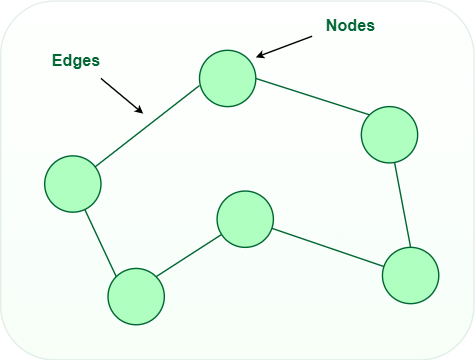Graph Data Structure

Graph Representation

In the graph data structure, a graph representation is a technique to store graphs in the memory of the computer. There are many ways to represent a graph:

The following two are the most commonly used representations of a graph.

1. Adjacency Matrix: An adjacency matrix represents a graph as a matrix of boolean values (0s and 1s). In a computer, a finite graph can be represented as a square matrix, where the boolean value indicates if two vertices are connected directly.
2. Adjacency List: An adjacency list represents a graph as an array of linked lists where an index of the array represents a vertex and each element in its linked list represents the other vertices that are connected with the edges, or say its neighbor.

Graph Operations:

• Check if the graph contains a given value.
• Find the path from one vertex to another vertex.

Below is the implementation of Graph in javascript:

## Javascript

 `// create a graph class``class Graph {``    ``// defining vertex array and``    ``// adjacent list``    ``constructor(noOfVertices)``    ``{``        ``this``.noOfVertices = noOfVertices;``        ``this``.AdjList = ``new` `Map();``    ``}` `    ``// functions to be implemented` `  ``// add vertex to the graph``addVertex(v)``{``    ``// initialize the adjacent list with a``    ``// null array``    ``this``.AdjList.set(v, []);``}` `    ``// add edge to the graph``addEdge(v, w)``{``    ``// get the list for vertex v and put the``    ``// vertex w denoting edge between v and w``    ``this``.AdjList.get(v).push(w);` `    ``// Since graph is undirected,``    ``// add an edge from w to v also``    ``this``.AdjList.get(w).push(v);``}` `// Prints the vertex and adjacency list``printGraph()``{``    ``// get all the vertices``    ``var` `get_keys = ``this``.AdjList.keys();` `    ``// iterate over the vertices``    ``for` `(``var` `i of get_keys)``{``        ``// get the corresponding adjacency list``        ``// for the vertex``        ``var` `get_values = ``this``.AdjList.get(i);``        ``var` `conc = ``""``;` `        ``// iterate over the adjacency list``        ``// concatenate the values into a string``        ``for` `(``var` `j of get_values)``            ``conc += j + ``" "``;` `        ``// print the vertex and its adjacency list``        ``console.log(i + ``" -> "` `+ conc);``    ``}``}``}` ` `  `// Using the above implemented graph class``var` `g = ``new` `Graph(6);``var` `vertices = [ ``'A'``, ``'B'``, ``'C'``, ``'D'``, ``'E'``, ``'F'` `];` `// adding vertices``for` `(``var` `i = 0; i < vertices.length; i++) {``    ``g.addVertex(vertices[i]);``}` `// adding edges``g.addEdge(``'A'``, ``'B'``);``g.addEdge(``'A'``, ``'D'``);``g.addEdge(``'A'``, ``'E'``);``g.addEdge(``'B'``, ``'C'``);``g.addEdge(``'D'``, ``'E'``);``g.addEdge(``'E'``, ``'F'``);``g.addEdge(``'E'``, ``'C'``);``g.addEdge(``'C'``, ``'F'``);` `// prints all vertex and``// its adjacency list``// A -> B D E``// B -> A C``// C -> B E F``// D -> A E``// E -> A D F C``// F -> E C``g.printGraph();`

Output

```A -> B D E
B -> A C
C -> B E F
D -> A E
E -> A D F C
F -> E C ```

## 3. Practice Problems on Data Structures and Algorithms (DSA)

For practicing problems on individual data structures and algorithms, you can use the following links:

Apart from these, there are many other practice problems that you can refer based on their respective difficulties:

You can also try to solve the most asked interview questions based on the list curated by us at:

You can also try our curated lists of problems below articles: